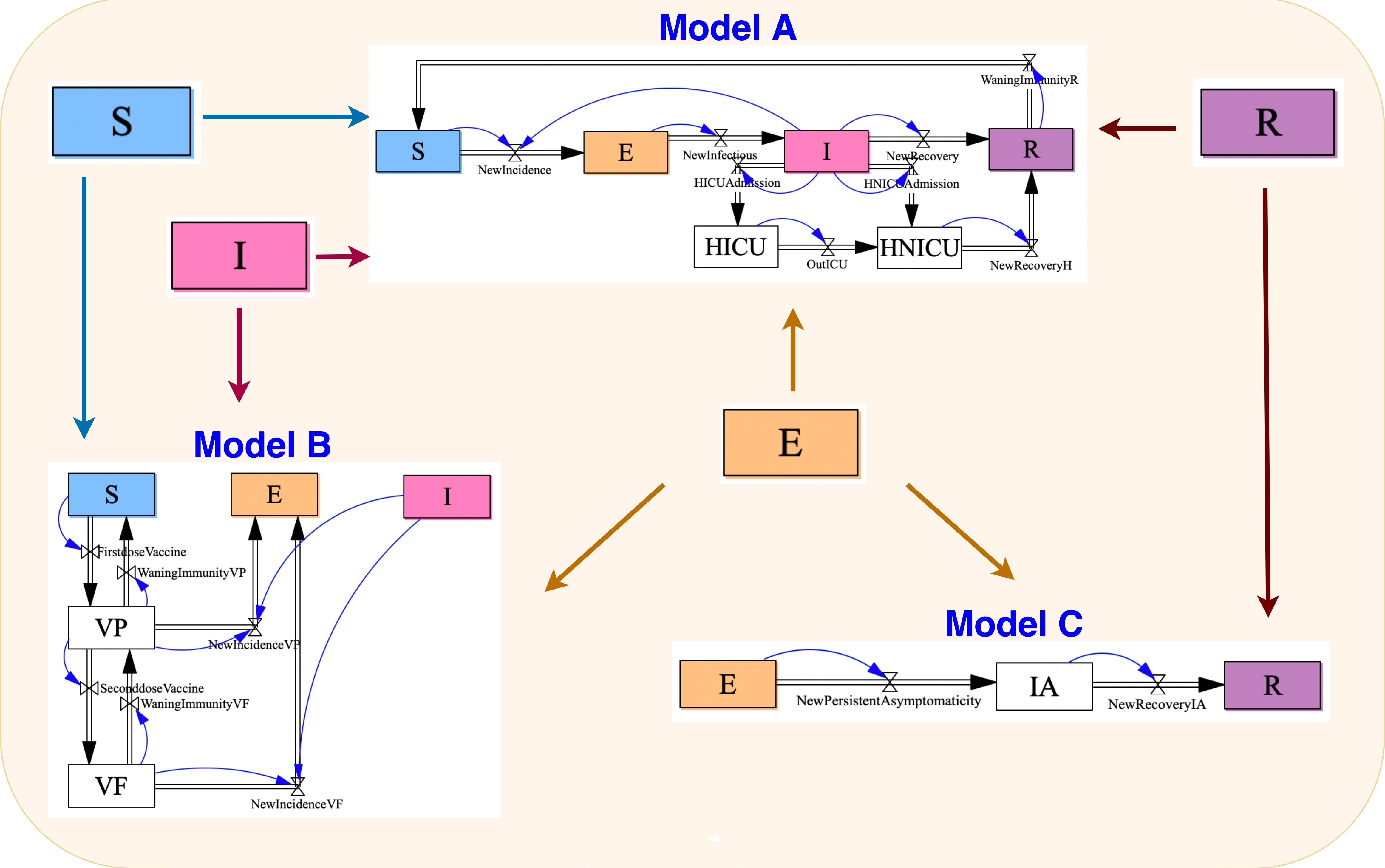#### Theoretical Computer Science Seminar, University of Birmingham, October 20, 2023### Software for Compositional Modeling

Mathematical models of disease are important and widely used, but building and working with these models at scale is challenging. Many epidemiologists use “stock and flow diagrams” to describe ordinary differential equation (ODE) models of disease dynamics. This talk introduces the mathematics of stock and flow diagrams and two software tools for working with them. The first, called StockFlow.jl, is based on category theory and written in AlgebraicJulia. The second, called ModelCollab, runs on a web browser and serves as a graphical user interface for StockFlow.jl. Modelers often regard diagrams as an informal step toward a mathematically rigorous formulation of a model in terms of ODEs. However, stock and flow diagrams have a precise mathematical syntax. Formulating this syntax using category theory has many advantages, but I will focus on three: functorial semantics, model composition, and model stratification. This is joint work with Xiaoyan Li, Sophie Libkind, Nathaniel Osgood, Evan Patterson and Eric Redekopp.

You can see my slides here and watch my talk here:

You can get the code for Stockflow here and ModelCollab here.